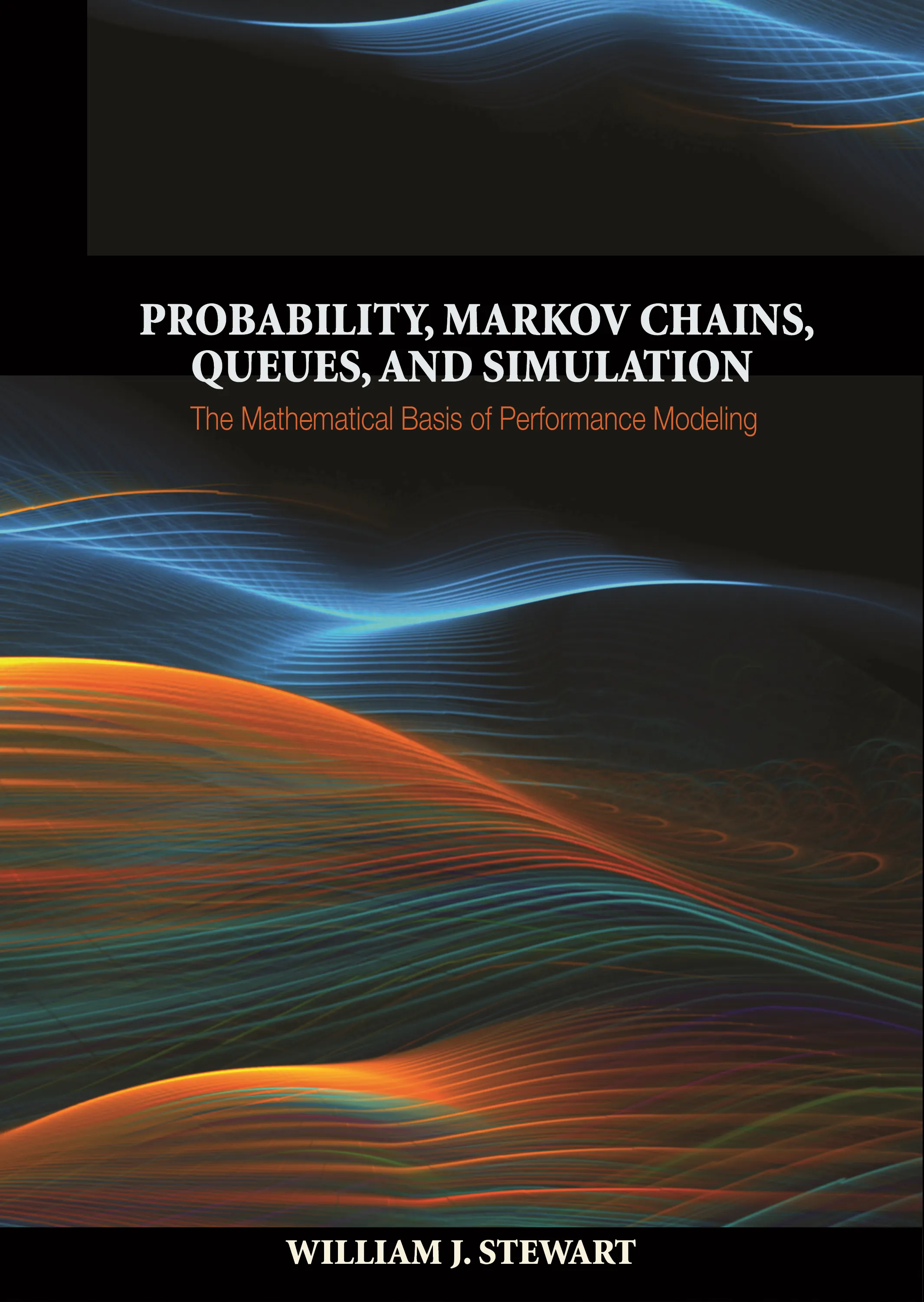#### Probability, Markov Chains, Queues, and Simulation

William J. Stewart

##### ebooks

Our ebooks editions are available from these online vendors:

Many of our ebooks are available through library electronic resources including these platforms:

# Probability, Markov Chains, Queues, and Simulation: The Mathematical Basis of Performance Modeling### Hardcover

Price:
\$120.00 / £100.00
ISBN:
Published:
07/26/2009
2009
Pages:
776
Size:
7 x 10 in.
Illus:
175 line illus.

Probability, Markov Chains, Queues, and Simulation provides a modern and authoritative treatment of the mathematical processes that underlie performance modeling. The detailed explanations of mathematical derivations and numerous illustrative examples make this textbook readily accessible to graduate and advanced undergraduate students taking courses in which stochastic processes play a fundamental role. The textbook is relevant to a wide variety of fields, including computer science, engineering, operations research, statistics, and mathematics.

The textbook looks at the fundamentals of probability theory, from the basic concepts of set-based probability, through probability distributions, to bounds, limit theorems, and the laws of large numbers. Discrete and continuous-time Markov chains are analyzed from a theoretical and computational point of view. Topics include the Chapman-Kolmogorov equations; irreducibility; the potential, fundamental, and reachability matrices; random walk problems; reversibility; renewal processes; and the numerical computation of stationary and transient distributions. The M/M/1 queue and its extensions to more general birth-death processes are analyzed in detail, as are queues with phase-type arrival and service processes. The M/G/1 and G/M/1 queues are solved using embedded Markov chains; the busy period, residual service time, and priority scheduling are treated. Open and closed queueing networks are analyzed. The final part of the book addresses the mathematical basis of simulation.

Each chapter of the textbook concludes with an extensive set of exercises. An instructor’s solution manual, in which all exercises are completely worked out, is also available (to professors only).

• Numerous examples illuminate the mathematical theories

• Carefully detailed explanations of mathematical derivations guarantee a valuable pedagogical approach

• Each chapter concludes with an extensive set of exercises